A quantile-quantile plot (Q-Q plot) is a graphical tool that compares a data distribution and a specified probability distribution. If the points in a Q-Q plot appear to fall on a straight line, that is evidence that the data can be approximately modeled by the target distribution. Although it is not necessary, some data analysts like to overlay a reference line to help "guide their eyes" as to whether the values in the plot fall on a straight line. This article describes three ways to overlay a reference line on a Q-Q plot. The first two lines are useful during the exploratory phase of data analysis; the third line visually represents the estimates of the location and scale parameters in the fitted model distribution. The three lines are:

• A line that connect the 25th and 75th percentiles of the data and reference distributions
• A least squares regression line
• A line whose intercept and slope are determined by maximum likelihood estimates of the location and scale parameters of the target distribution.

If you need to review Q-Q plots, see my previous article that describes what a Q-Q plot is, how to construct a Q-Q plot in SAS, and how to interpret a Q-Q plot.

### Create a basic Q-Q plot in SAS

Let me be clear: It is not necessary to overlay a line on a Q-Q plot. You can display only the points on a Q-Q plot and, in fact, that is the default behavior in SAS when you create a Q-Q plot by using the QQPLOT statement in PROC UNIVARIATE.

The following DATA step generates 97 random values from an exponential distribution with shape parameter σ = 2 and three artificial "outliers." The call to PROC UNIVARIATE creates a Q-Q plot, which is shown:

```data Q(keep=y); call streaminit(321); do i = 1 to 97; y = round( rand("Expon", 2), 0.001); /* Y ~ Exp(2), rounded to nearest 0.001 */ output; end; do y = 10,11,15; output; end; /* add outliers */ run;   proc univariate data=Q; qqplot y / exp grid; /* plot data quantiles against Exp(1) */ ods select QQPlot; ods output QQPlot=QQPlot; /* for later use: save quantiles to a data set */ run;```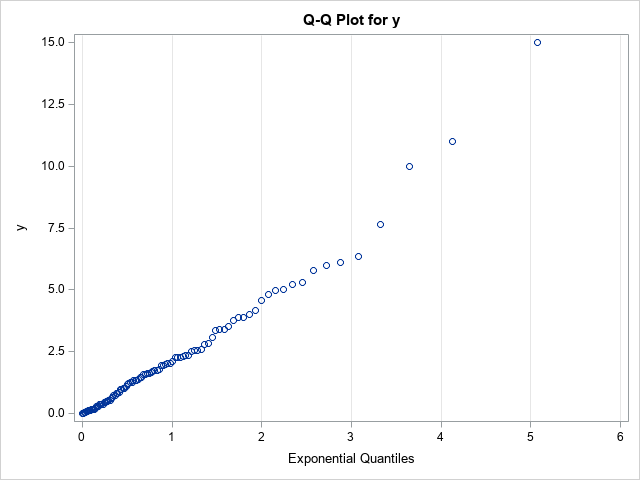The vertical axis of the Q-Q plot displays the sorted values of the data; the horizontal axis displays evenly spaced quantiles of the standardized target distribution, which in this case is the exponential distribution with scale parameter σ = 1. Most of the points appear to fall on a straight line, which indicates that these (simulated) data might be reasonably modeled by using an exponential distribution. The slope of the line appears to be approximately 2, which is a crude estimate of the scale parameter (σ). The Y-intercept of the line appears to be approximately 0, which is a crude estimate of the location parameter (the threshold parameter, θ).

Although the basic Q-Q plot provides all the information you need to decide that these data can be modeled by an exponential distribution, some data sets are less clear. The Q-Q plot might show a slight bend or wiggle, and you might want to overlay a reference line to assess how severely the pattern deviates from a straight line. The problem is, what line should you use?

### A reference line for the Q-Q plot

Cleveland (Visualiizing Data, 1993, p. 31) recommends overlaying a line that connects the first and third quartiles. That is, let p25 and p75 be the 25th and 75th percentiles of the target distribution, respectively, and let y25 and y75 be the 25th and 75th percentiles of the ordered data values. Then Cleveland recommends plotting the line through the ordered pairs (p25, y25) and (p75, yy5).

In SAS, you can use PROC MEANS to compute the 25th and 75th percentiles for the X and Y variables in the Q-Q plot. You can then use the DATA step or PROC SQL to compute the slope of the line that passes between the percentiles. The following statements analyze the Q-Q plot data that was created by using the ODS OUTPUT statement in the previous section:

```proc means data=QQPlot P25 P75; var Quantile Data; /* ODS OUTPUT created the variables Quantile (X) and Data (Y) */ output out=Pctl P25= P75= / autoname; run;   data _null_; set Pctl; slope = (Data_P75 - Data_P25) / (Quantile_P75 - Quantile_P25); /* dy / dx */ /* if desired, put point-slope values into macro variables to help plot the line */ call symputx("x1", Quantile_P25); call symputx("y1", Data_P25); call symput("Slope", putn(slope,"BEST5.")); run;   title "Q-Q Plot with Reference Line"; title2 "Reference Line through First and Third Quartiles"; title3 "Slope = &slope"; proc sgplot data=QQPlot; scatter x=Quantile y=Data; lineparm x=&x1 y=&y1 slope=&slope / lineattrs=(color=Green) legendlabel="Percentile Estimate"; xaxis grid label="Exponential Quantiles"; yaxis grid; run;```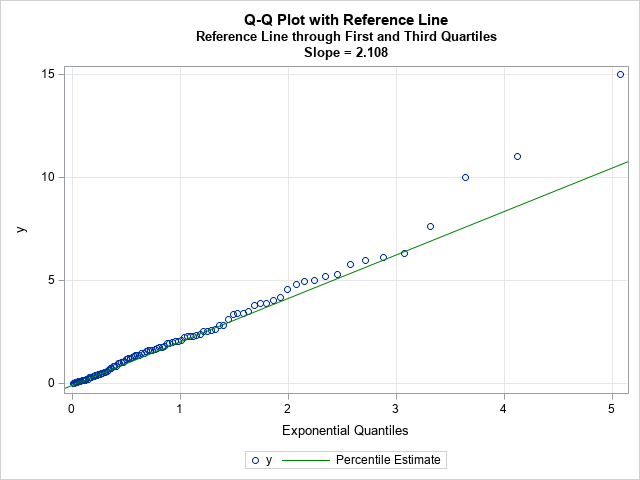Because the line passes through the first and third quartiles, the slope of the line is robust to outliers in the tails of the data. The line often provides a simple visual guide to help you determine whether the central portion of the data matches the quantiles of the specified probability distribution.

Keep in mind that this is a visual guide. The slope and intercept for this line should not be used as parameter estimates for the location and scale parameters of the probability distribution, although they could be used as an initial guess for an optimization that estimates the location and scale parameters for the model distribution.

### Regression lines as visual guides for a Q-Q plot

Let's be honest, when a statistician sees a scatter plot for which the points appear to be linearly related, there is a Pavlovian reflex to fit a regression line to the values in the plot. However, I can think of several reasons to avoid adding a regression line to a Q-Q plot:

• The values in the Q-Q plot do not satisfy the assumptions of ordinary least squares (OLS) regression. For example, the points are not a random sample and there is no reason to assume that the errors in the Y direction are normally distributed.
• In practice, the tails of the probability distribution rarely match the tails of the data distribution. In fact, the points to the extreme left and right of a Q-Q plot often exhibit a systematic bend away from a straight line. In an OLS regression, these extreme points will be high-leverage points that will unduly affect the OLS fit.

If you choose to ignore these problems, you can use the REG statement in PROC SGPLOT to add a reference line. Alternatively, you can use PROC REG in SAS (perhaps with the NOINT option if the location parameter is zero) to obtain an estimate of the slope:

```proc reg data=QQPlot plots=NONE; model Data = Quantile / NOINT; /* use NOINT when location parameter is 0 */ ods select ParameterEstimates; quit;   title2 "Least Squares Reference Line"; proc sgplot data=QQPlot; scatter x=Quantile y=Data; lineparm x=0 y=0 slope=2.36558 / lineattrs=(color=Red) legendlabel="OLS Estimate"; xaxis grid label="Exponential Quantiles"; yaxis grid; run;```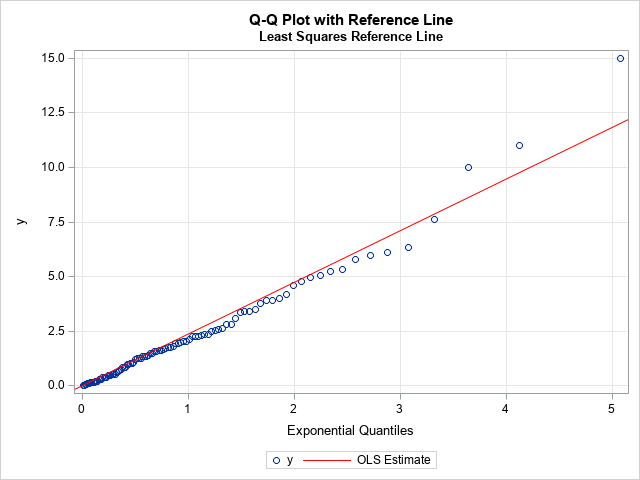For these data, I used the NOINT option to set the threshold parameter to 0. The zero-intercept line with slope 2.36558 is overlaid on the Q-Q plot. As expected, the outliers in the upper-right corner of the Q-Q plot have pulled the regression line upward, so the regression line has a steeper slope than the reference line based on the first and third quartiles. Because the tails of an empirical distribution often differ from the tails of the target distribution, the regression-based reference line can be misleading. I do not recommend its use.

### Maximum likelihood estimates

The previous sections describe two ways to overlay a reference line during the exploratory phase of the data analysis. The purpose of the reference line is to guide your eye and help you determine whether the points in the Q-Q plot appear to fall on a straight line. If so, you can move to the modeling phase.

In the modeling phase, you use a parameter estimation method to fit the parameters in the target distribution. Maximum likelihood estimation (MLE) is often the method-of-choice for estimating parameters from data. You can use the HISTOGRAM statement in PROC UNIVARIATE to obtain a maximum likelihood estimate of the shape parameter for the exponential distribution, which turns out to be 2.21387. If you specify the location and scale parameters in the QQPLOT statement, PROC UNIVARIATE will automatically overlay a line that represents that fitted values:

```proc univariate data=Q; histogram y / exp; qqplot y / exp(threshold=0 scale=est) odstitle="Q-Q Plot with MLE Estimate" grid; ods select ParameterEstimates GoodnessOfFit QQPlot; run;```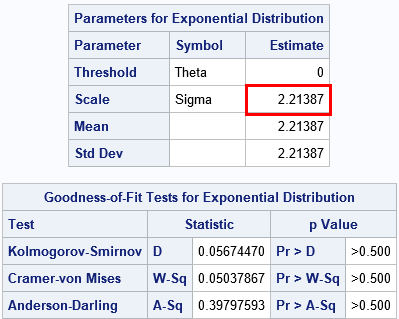The ParameterEstimates table shows the maximum likelihood estimate. The GoodnessOfFit table shows that there is no evidence to reject the hypothesis that these data came from an Exp(σ=2.21) distribution.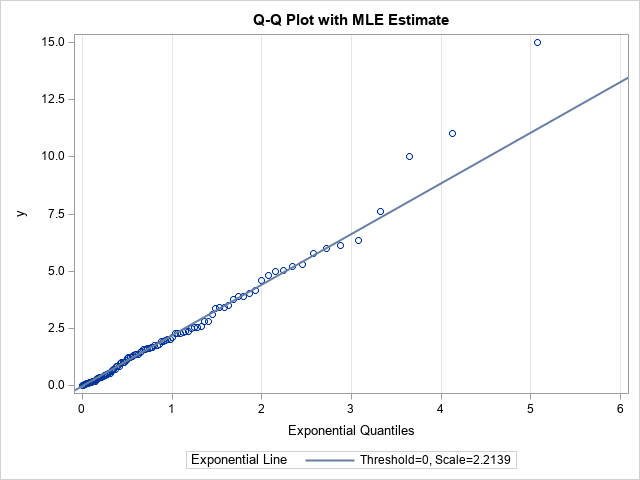Notice the distinction between this line and the previous lines. This line is the result of fitting the target distribution to the data (MLE) whereas the previous lines were visual guides. When you display a Q-Q plot that has a diagonal line, you should state how the line was computed.

In conclusion, you can display a Q-Q plot without adding any reference line. If you choose to overlay a line, there are three common methods. During the exploratory phase of analysis, you can display a line that connects the 25th and 75th percentiles of the data and target distributions. (Some practitioners use an OLS regression line, but I do not recommend it.) During the modeling phase, you can use maximum likelihood estimation or some other fitting method to estimate the location and scale of the target distribution. Those estimates can be used as the intercept and slope, respectively, of a line on the Q-Q plot. PROC UNIVARIATE in SAS displays this line automatically when you fit a distribution.

The post Three ways to add a line to a Q-Q plot appeared first on The DO Loop.When you overlay two series in PROC SGPLOT, you can either plot both series on the same axis or you can assign one series to the main axis (Y) and another to a secondary axis (Y2). If you use the Y and Y2 axes, they are scaled independently by default, which is usually what you want. However, if the measurements for the two series are linearly related to each other, then you might want to specify the tick values for the Y2 axis so that they align with the corresponding tick marks for the Y axis. This article shows how to align the Y and Y2 axes in PROC SGPLOT in SAS for two common situations.

### Different scales for one set of measurements

The simplest situation is a single set of data that you want to display in two different units. For example, you might use one axis to display the data in imperial units (pounds, gallons, degrees Fahrenheit, etc.) and the other axis to display the data in metric units (kilograms, liters, degrees Celsius, etc.).

To plot the data, define one variable for each unit. For example, the Sashelp.Class data records the weight for 19 students in pounds. The following DATA view creates a new variable that records the same data in kilograms. The subsequent call to PROC SGPLOT plots the pounds on the Y axis (left axis) and the kilograms on the Y2 axis (right axis). However, as you will see, there is a problem with the default scaling of the two axes:

```data PoundsKilos / view=PoundsKilos; set Sashelp.Class(rename=(Weight=Pounds)); Kilograms = 0.453592 * Pounds; /* convert pounds to kilos */ run;   title "Independent Axes"; title2 "Markers Do Not Align Correctly!"; /* the tick marks on each axis are independent */ proc sgplot data=PoundsKilos; scatter x=Height y=Pounds; scatter x=Height y=Kilograms / Y2Axis; run;```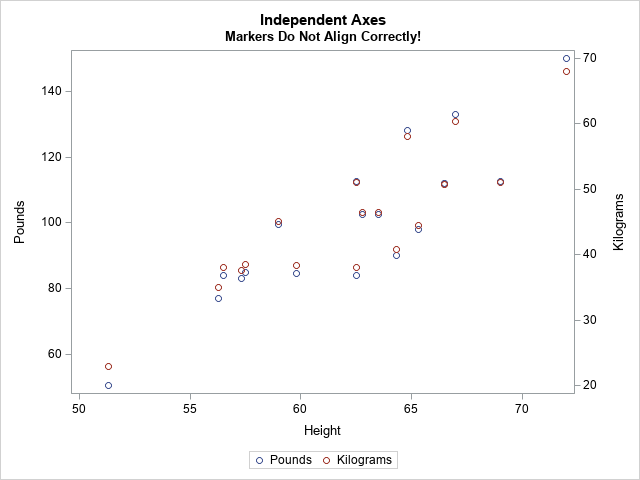The markers for the kilogram measurements should exactly overlap the markers for pounds, but they don't. The Y and Y2 axes are independently scaled because PROC SGPLOT does not know that pounds and kilograms are linearly related. The SGPLOT procedure displays each variable by using a range of round numbers (multiples of 10 or 20). The range for the Y2 axis is [20, 70] kilograms, which corresponds to a range of [44.1, 154.3] pounds. However, the range for the Y axis is approximately [50, 150] pounds. Because the axes display different ranges, the markers do not overlap.

To improve this graph, use the VALUES= and VALUESDISPLAY= options on the YAXIS statement (or Y2AXIS statement) to force the ticks marks on one axis to align with the corresponding tick marks on the other axis. In the following DATA step, I use the kilogram scale as the standard and compute the corresponding pounds.

```data Ticks; do Kilograms = 20 to 70 by 10; /* for each Y2 tick */ Pounds = Kilograms / 0.453592; /* convert kilos to pounds */ Approx = round(Pounds, 0.1); /* use rounded values to display tick values */ output; end; run; proc print; run;```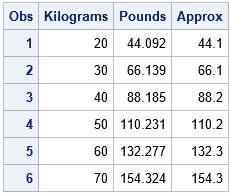You can use the Pounds column in the table to set the VALUES= list on the YAXIS statement. You can use the Approx column to set the VALUESDISPLAY= list, as follows:

```/* align tick marks on each axis */ title "Both Axes Use the Same Scale"; proc sgplot data=PoundsKilos noautolegend; scatter x=Height y=Pounds; /* Make sure the plots overlay exactly! Then you can set SIZE=0 */ scatter x=Height y=Kilograms / markerattrs=(size=0) Y2Axis; yaxis grid values=(44.092 66.139 88.185 110.231 132.277 154.324) valuesdisplay=('44.1' '66.1' '88.2' '110.2' '132.3' '154.3'); run;```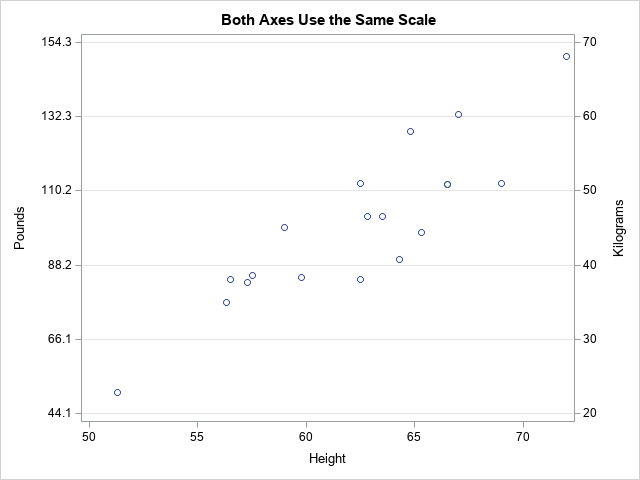Success! The markers for the two variables align exactly. After verifying that they align, you can use the MARKERATTRS=(SIZE=0) option to suppress the display of one of the markers.

Notice that the Y axis (pounds) no longer displays "nice numbers" because I put the tick marks at the same vertical heights on both axes. A different way to solve the misalignment problem is to use the MIN=, MAX=, THRESHOLDMIN=, and THRESHOLDMAX= options on both axes. This will enable both axes to use "nice numbers" while still aligning the data. If you want to try this approach, here are the YAXIS and Y2AXIS statements:

``` /* set the axes ranges to coresponding values */ yaxis grid thresholdmin=0 thresholdmax=0 min=44.1 max=154.3; y2axis grid thresholdmin=0 thresholdmax=0 min=20 max=70;```

### Different scales for different measurements

Another situation that requires two Y axes is the case of two series that use different units. For example, you might want to plot the revenue for a US company (in dollars) and the revenue for a Japanese company (in yen) for a certain time period. You can use the conversion rate between yen and dollars to align the values on the axes. Of course, the conversion from Japanese yen to the US dollars changes each day, but you can use an average conversion rate to set the correspondence between the axes.

This situation also occurs when two devices use different methods to measure the same quantity. The following example shows measurements for a patient who receives a certain treatment. The quantity of a substance in the patient's blood is measured at baseline and for every hour thereafter. The quantity is measured in two ways: by using a traditional blood test and by using a new noninvasive device that measures electrical impedance. The following statements define and plot the data. The two axes are scaled by using the default method:

```data BloodTest1; label t="Hours after Medication" x="micrograms per deciliter" y="kiloOhms"; input x y @@; t = _N_ - 1; datalines; 169.0 45.5 130.8 33.4 109.0 23.8 94.1 19.8 86.3 20.4 78.4 18.7 76.1 16.1 72.2 16.7 70.0 11.9 69.8 14.6 69.5 10.6 68.7 12.7 67.3 16.9 ;   title "Overlay Measurements for Two Medical Devices"; title2 "Default Scaling"; proc sgplot data=BloodTest1; series x=t y=x / markers legendlabel="Standard Lab Value"; series x=t y=y / markers Y2Axis legendlabel="New Device"; xaxis values=(0 to 12 by 2); yaxis grid label="micrograms per deciliter"; y2axis grid label="kiloOhms"; run;```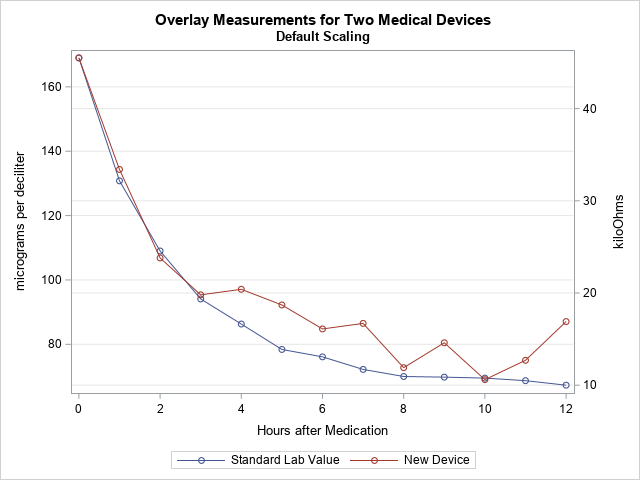In this graph, the Y axes are scaled independently. However, the company that manufactures the device used Deming regression to establish that the measurements from the two devices are linearly related by the equation Y = –10.56415 + 0.354463*X, where X is the measurement from the blood test. You can use this linear equation to set the scales for the two axes.

The following DATA step uses the Deming regression estimates to convert the tick marks on the Y axis into values for the Y2 axis. (Click here for the PROC PRINT output.) The call to PROC SGPLOT creates a graph in which the Y2 axis is aligned with the Y axis according to the Deming regression estimates.

```data Ticks; do Y1 = 60 to 160 by 20; /* use Deming regression to find one set of ticks in terms of the other */ Y2 = -10.56415 + 0.354463 * Y1; /* kiloOhms as a function of micrograms/dL */ Approx = round(Y2, 0.1); output; end; run;   proc print; run;   title "Align Y Axes for Different Series"; title2 "Measurements are Linearly Related"; proc sgplot data=BloodTest1; series x=t y=x / markers legendlabel="Standard Lab Value"; series x=t y=y / markers Y2Axis legendlabel="New Device"; xaxis values=(0 to 12 by 2); yaxis grid label="micrograms per deciliter" offsetmax=0.1 values=(60 to 160 by 20); /* the same offsets must be used in both YAXIS and Y2AXIS stmts */ y2axis grid label="kiloOhms" offsetmax=0.1 values=(10.7036 17.7929 24.8822 31.9714 39.0607 46.1499) valuesdisplay=('10.7' '17.8' '24.9' '32.0' '39.1' '46.1'); run;```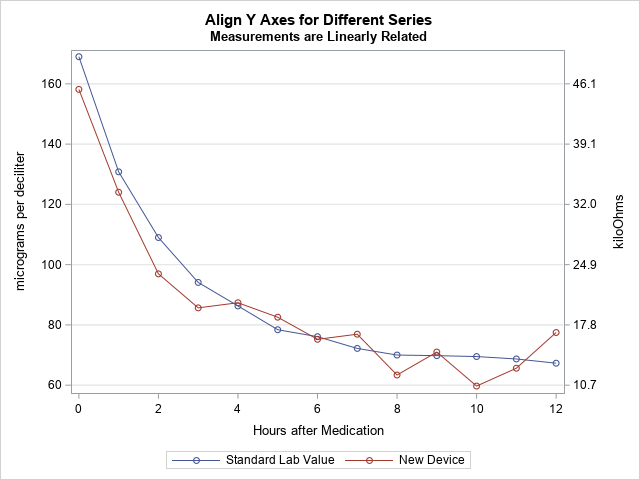In this new graph, the measurements are displayed on compatible scales and the reference lines connect round numbers on one axis to the corresponding values on the other axis.

The post How to align the Y and Y2 axes in PROC SGPLOT appeared first on The DO Loop.Numbers don't lie, but sometimes they don't reveal the full story. Last week I wrote about the most popular articles from The DO Loop in 2018. The popular articles are inevitably about elementary topics in SAS programming or statistics because those topics have broad appeal. However, I also write about advanced topics, which are less popular but fill an important niche in the SAS community. Not everyone needs to know how to fit a Pareto distribution in SAS or how to compute distance-based measures of correlation in SAS. Nevertheless, these topics are interesting to think about.

I believe that learning should not stop when we leave school. If you, too, are a lifelong learner, the following topics deserve a second look. I've included articles from four different categories.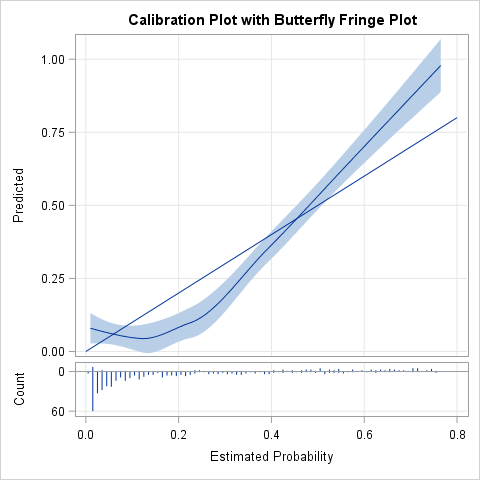### Random numbers and resampling methods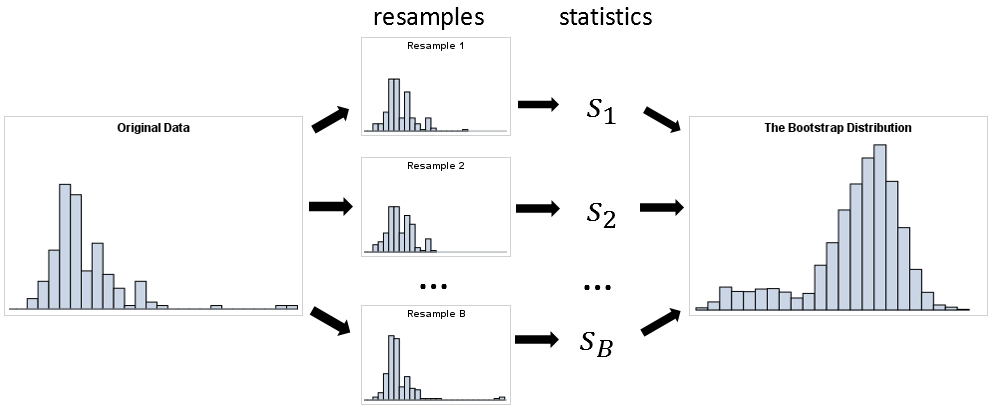### Optimization

These articles are technical but provide tips and techniques that you might find useful. Choose a few topics that are unfamiliar and teach yourself something new in this New Year!

Do you have a favorite article from 2018 that I did not include on the list? Share it in a comment!

The post 10 posts from 2018 that deserve a second look appeared first on The DO Loop.Deming regression (also called errors-in-variables regression) is a total regression method that fits a regression line when the measurements of both the explanatory variable (X) and the response variable (Y) are assumed to be subject to normally distributed errors. Recall that in ordinary least squares regression, the explanatory variable (X) is assumed to be measured without error. Deming regression is explained in a Wikipedia article and in a paper by K. Linnet (1993).

A situation in which both X and Y are measured with errors arises when comparing measurements from different instruments or medical devices. For example, suppose a lab test measures the amount of some substance in a patient's blood. If you want to monitor this substance at regular intervals (for example, hourly), it is expensive, painful, and inconvenient to take the patient's blood multiple times. If someone invents a medical device that goes on the patient's finger and measures the substance indirectly (perhaps by measuring an electrical property such as bioimpedance), then that device would be an improved way to monitor the patient. However, as explained in Deal, Pate, and El Rouby (2009), the FDA would first need to approve the device and determine that it measures the response as accurately as the existing lab test. The FDA encourages the use of Deming regression for method-comparison studies.

### Deming regression in SAS

There are several ways to compute a Deming Regression in SAS. The SAS FASTats site suggests maximum likelihood estimation (MLE) by using PROC OPTMODEL, PROC IML, or PROC NLMIXED. However, you can solve the MLE equations explicitly to obtain an explicit formula for the regression estimates. Deal, Pate, and El Rouby (2009) present a rather complicated macro, whereas Njoya and Hemyari (2017) use simple SQL statements. Both authors also provide SAS code for estimating the variance of the Deming regression estimates, either by using the jackknife method or by using the bootstrap. However, the resampling schemes in both papers are inefficient because they use a macro loop to perform the jackknife or bootstrap.

The following SAS DATA Step defines pairs of hypothetical measurements for 65 patients, each of whom received the standard lab test (measured in micrograms per deciliter) and the new noninvasive device (measured in kiloohms):

```data BloodTest; label x="micrograms per deciliter" y="kiloOhms"; input x y @@; datalines; 169.0 45.5 130.8 33.4 109.0 23.8 94.1 19.8 86.3 20.4 78.4 18.7 76.1 16.1 72.2 16.7 70.0 11.9 69.8 14.6 69.5 10.6 68.7 12.7 67.3 16.9 174.7 57.8 137.9 39.0 114.6 30.4 99.8 21.1 90.1 21.7 85.1 25.2 80.7 20.6 78.1 19.3 77.8 20.9 76.0 18.2 77.8 18.3 74.2 15.7 73.1 13.9 182.5 55.5 144.0 38.7 123.8 35.1 107.6 30.6 96.9 25.7 92.8 19.2 87.2 22.4 86.3 18.4 84.4 20.7 83.7 20.6 83.3 20.0 83.9 18.8 82.7 21.8 160.8 49.9 122.7 32.2 102.6 19.2 86.6 14.7 76.1 16.6 69.6 18.8 66.7 7.4 64.4 8.2 63.0 15.5 61.7 13.7 61.2 9.2 62.4 12.0 58.4 15.2 171.3 48.7 136.3 36.1 111.9 28.6 96.5 21.8 90.3 25.6 82.9 16.8 78.1 14.1 76.5 14.2 73.5 11.9 74.4 17.7 73.9 17.6 71.9 10.2 72.0 15.6 ;   title "Deming Regression"; title2 "Gold Standard (X) vs New Method (Y)"; proc sgplot data=BloodTest noautolegend; scatter x=x y=y; lineparm x=0 y=-10.56415 slope=0.354463 / clip; /* Deming regression estimates */ xaxis grid label="Lab Test (micrograms per deciliter)"; yaxis grid label="New Device (kiloohms)"; run;```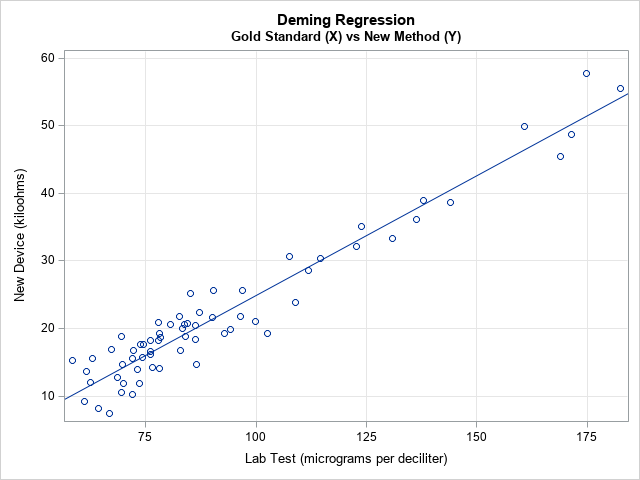The scatter plot shows the pairs of measurements for each patient. The linear pattern indicates that the new device is well calibrated with the standard lab test over a range of clinical values. The diagonal line represents the Deming regression estimate, which enables you to convert one measurement into another. For example, a lab test that reads 100 micrograms per deciliter is expected to correspond to 25 kiloohms on the new device and vice versa. (If you want to convert the new readings into the old, you can regress X onto Y and plot X on the vertical axis.)

The following SAS/IML function implements the explicit formulas that compute the slope and intercept of the Deming regression line:

```/* Deming Regression in SAS */ proc iml; start Deming(XY, lambda=); /* Equations from https://en.wikipedia.org/wiki/Deming_regression */ m = mean(XY); xMean = m; yMean = m; S = cov(XY); Sxx = S[1,1]; Sxy = S[1,2]; Syy = S[2,2]; /* if lambda is specified (eg, lambda=1), use it. Otherwise, estimate. */ if IsEmpty(lambda) then delta = Sxx / Syy; /* estimate of ratio of variance */ else delta = lambda; c = Syy - delta*Sxx; b1 = (c + sqrt(c**2 + 4*delta*Sxy**2)) / (2*Sxy); b0 = yMean - b1*xMean; return (b0 || b1); finish;   /* Test the program on the blood test data */ use BloodTest; read all var {x y} into XY; close; b = Deming(XY); print b[c={'Intercept' 'Slope'} L="Deming Regression"];```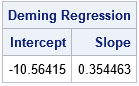The SAS/IML function can estimate the ratio of the variances of the X and Y variable. In the SAS macros by Deal, Pate, and El Rouby (2009) and Njoya and Hemyari (2017), the ratio is a parameter that is determined by the user. The examples in both papers use a ratio of 1, which assumes that the devices have an equal accuracy and use the same units of measurement. In the current example, the lab test and the electrical device use different units. The ratio of the variances for these hypothetical devices is about 7.4.

### Standard errors of estimates

You might wonder how accurate the parameter estimates are. Linnet (1993) recommends using the jackknife method to answer that question. I have previously explained how to jackknife estimates in SAS/IML, and the following program is copied from that article:

```/* Helper modules for jackknife estimates of standard error and CI for parameters: */ /* return the vector {1,2,...,i-1, i+1,...,n}, which excludes the scalar value i */ start SeqExclude(n,i); if i=1 then return 2:n; if i=n then return 1:n-1; return (1:i-1) || (i+1:n); finish;   /* return the i_th jackknife sample for (n x p) matrix X */ start JackSamp(X,i); return X[ SeqExclude(nrow(X), i), ]; /* return data without i_th row */ finish;   /* 1. Compute T = statistic on original data */ T = b;   /* 2. Compute statistic on each leave-one-out jackknife sample */ n = nrow(XY); T_LOO = j(n,2,.); /* LOO = "Leave One Out" */ do i = 1 to n; J = JackSamp(XY,i); T_LOO[i,] = Deming(J); end;   /* 3. compute mean of the LOO statistics */ T_Avg = mean( T_LOO );   /* 4. Compute jackknife estimates of standard error and CI */ stdErrJack = sqrt( (n-1)/n * (T_LOO - T_Avg)[##,] ); alpha = 0.05; tinv = quantile("T", 1-alpha/2, n-2); /* use df=n-2 b/c both x and y are estimated */ Lower = T - tinv#stdErrJack; Upper = T + tinv#stdErrJack; result = T` || T_Avg` || stdErrJack` || Lower` || Upper`; print result[c={"Estimate" "Mean Jackknife Estimate" "Std Error" "Lower 95% CL" "Upper 95% CL"} r={'Intercept' 'Slope'}];```The formulas for the jackknife computation differs slightly from the SAS macro by Deal, Pate, and El Rouby (2009). Because both X and Y have errors, the t quantile must be computed by using n–2 degrees of freedom, not n–1.

If X and Y are measured on the same scale, then the methods are well-calibrated when the 95% confidence interval (CI) for the intercept includes 0 and the CI for the intercept includes 1. In this example, the devices use different scales. The Deming regression line enables you to convert from one measurement scale to the other; the small standard errors (narrow CIs) indicate that this conversion is accurate.

In summary, you can use a simple set of formulas to implement Deming regression in SAS. This article uses SAS/IML to implement the regression estimates and the jackknife estimate of the standard errors. You can also use the macros that are mentioned in the section "Deming regression in SAS," but the macros are less efficient, and you need to specify the ratio of the variances of the data vectors.

The post Deming regression for comparing different measurement methods appeared first on The DO Loop.Last year, I wrote more than 100 posts for The DO Loop blog. Of these, the most popular articles were about data visualization, SAS programming tips, and statistical data analysis. Here are the most popular articles from 2018 in each category.

### Data Visualization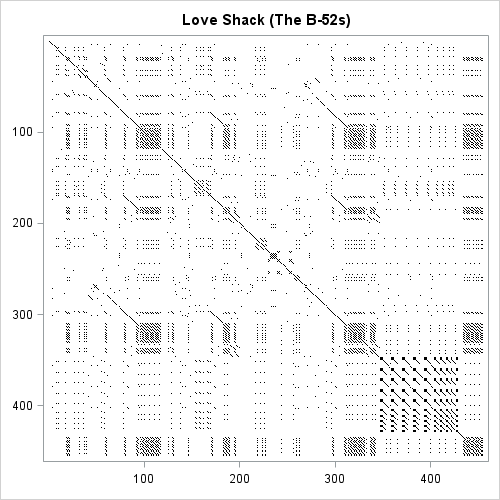### Statistics and Data Analysis

I write this blog because I love to learn new things and share what I know with others. If you want to learn something new, read (or re-read!) these popular articles from 2018. Then share this page with one of your colleagues. Happy New Year! I hope we both have many opportunities to learn and share in 2019!

The post Top posts from <em>The DO Loop</em> in 2018 appeared first on The DO Loop.I regularly see questions on a SAS discussion forum about how to visualize the predicted values for a mixed model that has at least one continuous variable, a categorical variable, and possibly an interaction term. SAS procedures such as GLM, GENMOD, and LOGISTIC can automatically produce plots of the predicted values versus the explanatory variables. These are called "fit plots" or "interaction plots" or "sliced fit plots." Although PROC MIXED does not automatically produce a "fit plot" for a mixed model, you can use the output from the procedure to construct a fit plot. In fact, two graphs are possible: one that incorporates the random effects for each subject in the predicted values and another that does not.

### Use PROC PLM to visualize the fixed-effect model

Because the MIXED (and GLIMMIX) procedure supports the STORE statement, you can write the model to an item store and then use the EFFECTPLOT statement in PROC PLM to visualize the predicted values. The resulting graph visualizes the fixed effects. The random effects are essentially "averaged out" or shown at their expected value, which is zero.

As an example, consider the following repeated measures example from the PROC MIXED documentation. The data are measurements for 11 girls and 16 boys recorded when the children were 8, 10, 12, and 14 years old. According to Pothoff and Roy (1964), "Each measurement is the distance, in millimeters, from the centre of the pituitary to the pteryomaxillary fissure. The reason why there is an occasional instance where this distance decreases with age is that the distance represents the relative position of two points." You can use a spaghetti plot to visualize the raw data:

```data pr; input Person Gender \$ y1 y2 y3 y4; y=y1; Age=8; output; y=y2; Age=10; output; y=y3; Age=12; output; y=y4; Age=14; output; drop y1-y4; label y="Relative Distance (mm)"; datalines; 1 F 21.0 20.0 21.5 23.0 2 F 21.0 21.5 24.0 25.5 3 F 20.5 24.0 24.5 26.0 4 F 23.5 24.5 25.0 26.5 5 F 21.5 23.0 22.5 23.5 6 F 20.0 21.0 21.0 22.5 7 F 21.5 22.5 23.0 25.0 8 F 23.0 23.0 23.5 24.0 9 F 20.0 21.0 22.0 21.5 10 F 16.5 19.0 19.0 19.5 11 F 24.5 25.0 28.0 28.0 12 M 26.0 25.0 29.0 31.0 13 M 21.5 22.5 23.0 26.5 14 M 23.0 22.5 24.0 27.5 15 M 25.5 27.5 26.5 27.0 16 M 20.0 23.5 22.5 26.0 17 M 24.5 25.5 27.0 28.5 18 M 22.0 22.0 24.5 26.5 19 M 24.0 21.5 24.5 25.5 20 M 23.0 20.5 31.0 26.0 21 M 27.5 28.0 31.0 31.5 22 M 23.0 23.0 23.5 25.0 23 M 21.5 23.5 24.0 28.0 24 M 17.0 24.5 26.0 29.5 25 M 22.5 25.5 25.5 26.0 26 M 23.0 24.5 26.0 30.0 27 M 22.0 21.5 23.5 25.0 ;   /* for information about the LEGENDITEM statement, see https://blogs.sas.com/content/iml/2018/02/12/merged-legends.html */ title "Relative Distance Between Features"; proc sgplot data=pr; series x=Age y=Y / group=Person groupLC=Gender curvelabel; /* LEGENDITEM is a SAS 9.4M5 feature. Delete the following statements in older versions of SAS */ legenditem type=line name="F" / label="Girls" lineattrs=GraphData1; legenditem type=line name="M" / label="Boys" lineattrs=GraphData2(pattern=dash); keylegend "M" "F"; run;```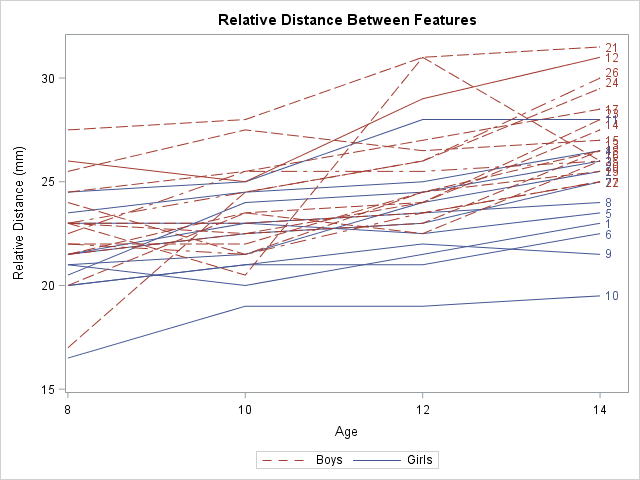Notice that I used the LEGENDITEM statement to customize the legend. The LEGENDITEM statement is a SAS 9.4M5 feature. You can delete the LEGENDITEM and KEYLEGEND statements to obtain the default legend.

One of the stated goals of the study is to model the average growth rate of the measurement for boys and for girls. You can see from the spaghetti plot that growth rate appears to be linear and that boys tend to have larger measurements than girls of the same age. However, it is not clear whether the rate (the slope of the average line) is the same for each gender or is significantly different.

The documentation example describes several ways to model the variance structure for the repeated measures. One choice is the AR(1) structure. The following statement uses the REPEATED statement to model the repeated measures. The STORE statement writes an item store that contains information about the model, which is used by PROC PLM to create effect plots:

```proc mixed data=pr method=ml; class Person Gender; model y = Gender Age Gender*Age / s; repeated / type=ar(1) sub=Person r; store out=MixedModel; /* create item store */ run;   proc plm restore=MixedModel; /* use item store to create fit plots */ effectplot fit(x=Age plotby=Gender); /* panel */ effectplot slicefit(x=Age sliceby=Gender); /* overlay */ *effectplot slicefit(x=Age sliceby=Person); /* ERROR: Person is not a fixed effect */ run;```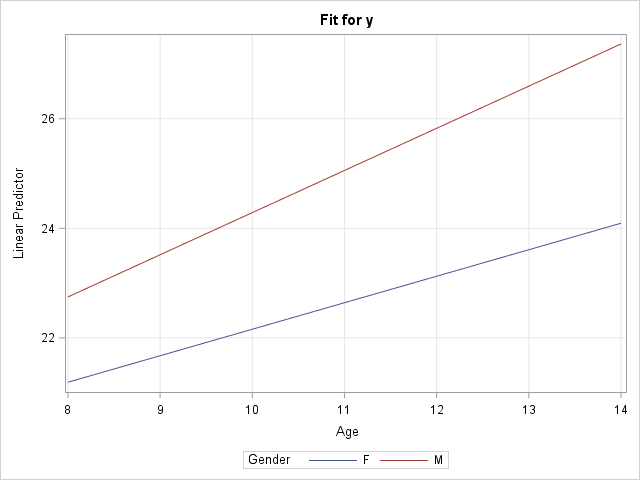The call to PROC PLM creates a panel of plots with confidence bands (not shown) and a graph that overlays the predicted values for males and females (shown). You can see a small difference in slopes between the two average growth curves, but the "Type 3 Tests of Fixed Effects" table from PROC MIXED (not shown) indicates that the difference is not statistically significant. The graph does not display the raw observations because PROC PLM does not have access to them. However, you can obtain a graph that overlays the observation by modifying the method in the next section.

Notice that this graph displays the model for boys and girls, but not for the individual subjects. In fact, I added a comment to the program that reminds you that it is an error to try to use the PERSON variable in the EFFECTPLOT statement because PERSON is not a fixed effect in the model. If you want to model to growth curves for individuals, see the next section.

### Use the OUTPRED= option visualize the random-coefficient model

The spaghetti plot seems to indicate that the growth curves for the individuals have the same slope but different intercepts. You can model this by using the RANDOM statement to add a random intercept effect to the model. The resulting graph "untangles" the spaghetti plot by plotting a line that best fits each individual's growth.

You can use the OUTPRED= option on the MODEL statement to create an output data set in which the predicted values incorporate the random intercept for each person:

```/* random coefficient model */ proc mixed data=pr method=ml; class Person Gender; model y = Gender Age Gender*Age / s outpred=Pred; random int / sub=Person; run;   /* BE SURE TO SORT by the X variable, before creating a SERIES plot. */ /* These data are already sorted, but the next line shows how to sort, if necessary */ proc sort data=Pred; by Gender Person Age; run;   title "Predicted Individual Growth Curves"; proc sgplot data=Pred; scatter x=Age y=Y / group=Gender; series x=Age y=Pred / group=Person GroupLC=Gender curvelabel; /* LEGENDITEM is a SAS 9.4M5 feature. Delete the following statements in older versions of SAS */ legenditem type=markerline name="F" / label="Girls" lineattrs=GraphData1 markerattrs=GraphData1; legenditem type=markerline name="M" / label="Boys" lineattrs=GraphData2(pattern=dash) markerattrs=GraphData2; keylegend "M" "F"; run;```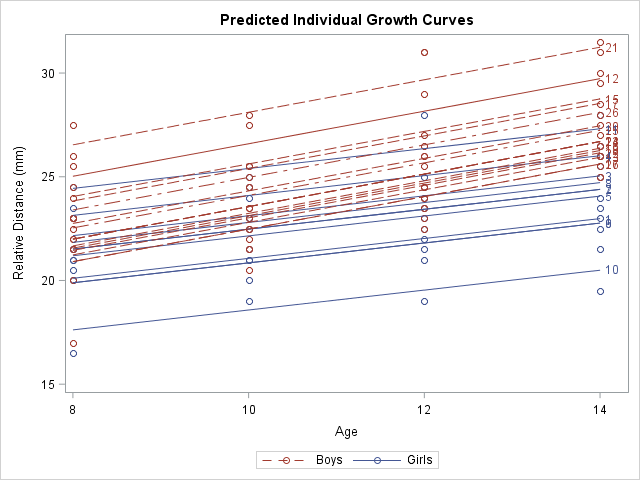This graph shows a smoothed version of the spaghetti plot. The graph enables you to see the variation in intercepts for the subjects but the slopes are determined by the gender of each individual. In statistical terms, the predicted values in this graph "incorporate the EBLUP values." The documentation contains the formula for the predicted values.

It is worth noting that the MODEL statement in PROC MIXED also supports an OUTPREDM= option. (The 'M' stands for 'marginal' model.) The OUTPREDM= option writes a data set that contains the predictions that do not "incorporate the EBLUP values", where EBLUP is an abbreviation for the "empirical best linear unbiased prediction." These are the same predicted values that you obtain from the STORE statement and PROC PLM. However, the OUTPREDM= data set gives you complete control over how you use the predicted values. For example, you can use the OUTPREDM= data set to add the original observations to the graph of the predicted values for boys and girls.

In summary, when you include a continuous covariate (like age) in a mixed model, there are two ways to visualize the "fit plot." You can use the STORE statement and PROC PLM to obtain a graph of the predictions from the "marginal model" that contains the fixed effects. (You can also use the OUTPREDM= option for this purpose.) Alternatively, if you are fitting a model that estimates random coefficients (intercepts or slopes), you can use the OUTPRED= option to write a data set that contains the predicted that incorporate the estimates.

The post Visualize a mixed model that has repeated measures or random coefficients appeared first on The DO Loop.Many data analysts use a quantile-quantile plot (Q-Q plot) to graphically assess whether data can be modeled by a probability distribution such as the normal, lognormal, or gamma distribution. You can use the QQPLOT statement in PROC UNIVARIATE to create a Q-Q plot for about a dozen common distributions. However, it can be useful to use a variant of the Q-Q plot called the probability plot, which enables you to graphically assess how well a model fits the tails of the data. A probability plot can also be created in PROC UNIVARIATE. It is essentially a Q-Q plot in which the X axis is labeled nonlinearly by using percentiles of the model distribution. This article describes how to create and interpret a probability plot in SAS.

### Use a probability plot to compare empirical and theoretical percentiles

When fitting a distribution by using maximum likelihood estimation or some other method, you might notice that the model fits well in the center of the data but not in the tails. This may or may not be a problem. If you want the model your "typical" customers or patients, the model might be useful even if it does not fit perfectly in the tails.

Goodness-of-fit (GOF) tests indicate how well the model fits the data everywhere. Deviations in the tail can cause a GOF test to reject the hypothesis that the model fits well. You can use a probability plot to determine the percentiles at which the model begins to deviate from the data.

The following example is from the PROC UNIVARIATE documentation. The data are the thickness of the copper plating of 100 circuit boards. To illustrate that a model might fail to fit the tails of the data, I have artificially created four fake outliers and appended them to the end of the data. The example fits a normal distribution to the data and creates two Q-Q plots and a probability plot:

```data Trans; input Thick @@; label Thick = 'Plating Thickness (mils)'; if _N_ <= 100 then Group="Real Data"; else Group = "Fake Data"; /* The last four observations are FAKE outliers */ datalines; 3.468 3.428 3.509 3.516 3.461 3.492 3.478 3.556 3.482 3.512 3.490 3.467 3.498 3.519 3.504 3.469 3.497 3.495 3.518 3.523 3.458 3.478 3.443 3.500 3.449 3.525 3.461 3.489 3.514 3.470 3.561 3.506 3.444 3.479 3.524 3.531 3.501 3.495 3.443 3.458 3.481 3.497 3.461 3.513 3.528 3.496 3.533 3.450 3.516 3.476 3.512 3.550 3.441 3.541 3.569 3.531 3.468 3.564 3.522 3.520 3.505 3.523 3.475 3.470 3.457 3.536 3.528 3.477 3.536 3.491 3.510 3.461 3.431 3.502 3.491 3.506 3.439 3.513 3.496 3.539 3.469 3.481 3.515 3.535 3.460 3.575 3.488 3.515 3.484 3.482 3.517 3.483 3.467 3.467 3.502 3.471 3.516 3.474 3.500 3.466 3.624 3.367 3.625 3.366 ;   title 'Analysis of Plating Thickness'; proc univariate data=Trans noprint; qqplot Thick / normal grid odstitle="Q-Q Plot"; qqplot Thick / normal PCTLAXIS(grid) odstitle="Q-Q Plot with PCTLAXIS Option"; probplot Thick / normal grid odstitle="Probability Plot"; run;```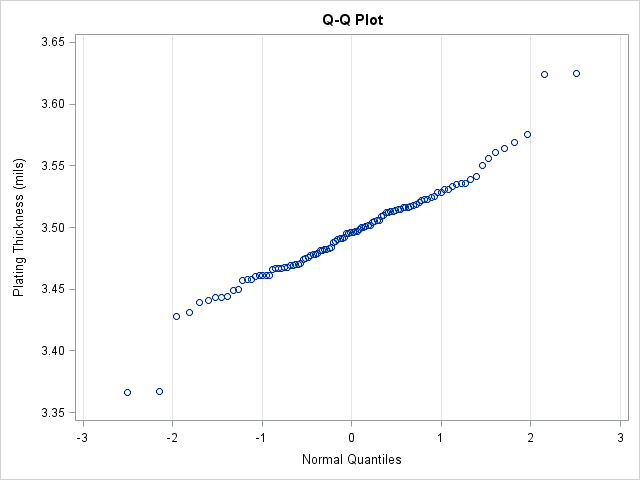The first Q-Q plot indicates whether the model (in this case, the normal distribution) fits the data. If the bulk of the data falls along a straight line, then there is evidence that the model fits. In this case, the model fits most of the data but not the four (artificial) points that I added.

If these were all real data, you might wrestle with whether you should accept the normal model or choose an alternative model. The Q-Q plot indicates that the normal data seems to fit the central portion of the data.

It would be useful if the plot displayed the percentiles of the normal distribution. The next image shows the Q-Q plot with the PCTLAXES option (in the background) and a probability plot (in the foreground). Notice that the positions of the markers are the same as for the Q-Q plot; only the labels on the axes have changed. The PCTLAXES option for the second QQPLOT statement creates a graph that displays percentiles of the normal distribution at the top of the plot. The probability plot eliminates the quantiles altogether and displays only the normal percentiles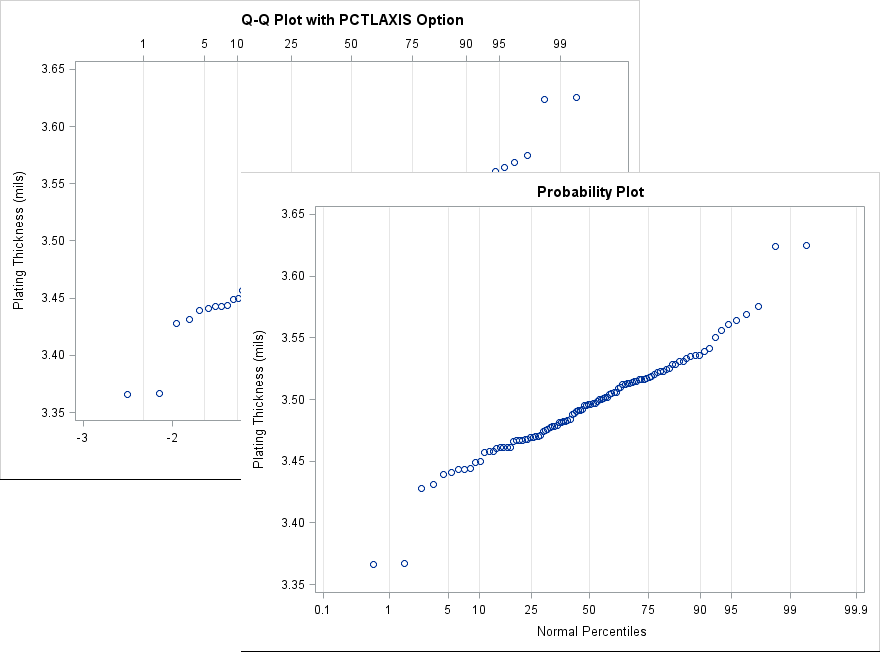By using the probability plot, you can estimate that the model fits well for the central 95% of the data. Only the upper and lower 2.5th tails of the model do not fit the data. If your goal is to model only the central 95% of the data, it might be fine to ignore the extreme data.

### Create a custom probability plot

I have previously shown how to construct a Q-Q plot from first principles. If you want to create a probability plot, you first create a Q-Q plot but then use the QUANTILE function to find the quantiles that correspond to the probabilities that you want to display on the axis. For example, the following SAS/IML statements print the quantiles for a set of typical probabilities:

```proc iml; p = {0.001 0.01 0.05 0.10 0.25 0.5 0.75 0.9 0.95 0.99 0.999}; qntl = quantile("Normal", p); /* compute tick locations */ print (qntl // 100*p)[r={"Quantiles" "Percentiles"} F=Best4.]; quit;```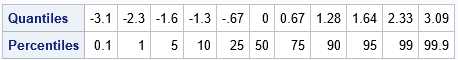You can use the VALUES= and VALUESDISPLAY= options on the XAXIS statement in PROC SGPLOT to display the probability values at the locations of the corresponding quantiles. The following DATA step creates the coordinates for a Q-Q plot but uses the previous table of quantile values to specify the values and labels on the X axis. This can be useful, for example, if you want to customize the probability plot. For example, the following call sets the colors and symbols of the markers, adds a legend, and sets the labels for the axes.

```proc sort data=Trans; by Thick; run;   data ProbPlot; set Trans nobs=nobs; y = Thick; /* for convenience, call variable Y */ v = (_N_ - 0.375) / (nobs + 0.25); /* Blom (1958) */ q = quantile("Normal", v); run;   title "Custom Probability Plot"; proc sgplot data=ProbPlot; scatter x=q y=y; yaxis grid label="Plating Thickness (mils)"; xaxis values=(-3.1 -2.3 -1.6 -1.3 -.67 0.00 0.67 1.28 1.64 2.33 3.09) valuesdisplay=('0.1' '1' '5' '10' '25' '50' '75' '90' '95' '99' '99.9') grid label="Normal Percentiles" fitpolicy=none; run;```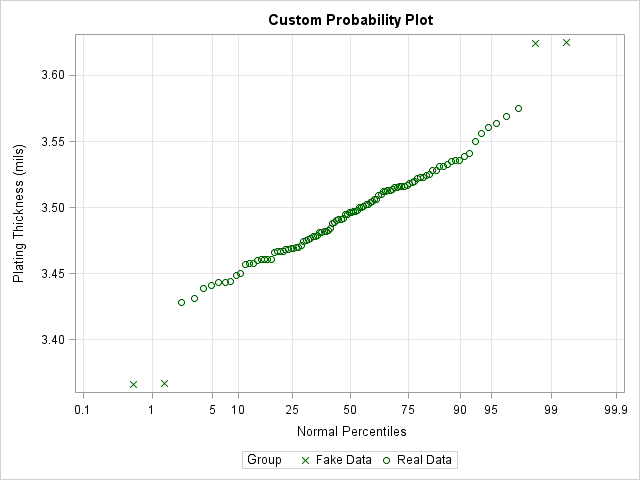The VALUES= and VALUESDISPLAY= options are very useful. I use them regularly to customize the location of tick marks and the values that are displayed at each tick.

In summary, you can use the QQPLOT statement (with the PCTLAXIS option) or the PROBPLOT statement in PROC UNIVARIATE to create a probability plot. A probability plot is essentially the same as a Q-Q plot except that the X axis displays the percentiles of a model distribution instead of quantiles. If you want additional customization (or want to examine a model that is not supported by PROC UNIVARIATE), then you can create the Q-Q plot manually. You can use the VALUES= and VALUESDISPLAY= options on the XAXIS statement in PROC SGPLOT to display the percentiles of the model distribution. For more about how to interpret a probability plot (especially for a non-normal reference distribution), see the PROC UNIVARIATE documentation.

The post Create a probability plot in SAS appeared first on The DO Loop.Recently a SAS programmer wanted to obtain a table of counts that was based on a histogram. I showed him how you can use the OUTHIST= option on the HISTOGRAM statement in PROC UNIVARIATE to obtain that information. For example, the following call to PROC UNIVARIATE creates a histogram for the MPG_City variable in the Sashelp.Cars data set. The histogram has 11 bins. The OUTHIST= option writes the counts for each bin to a SAS data set:

```proc univariate data=Sashelp.Cars noprint; var MPG_City; histogram MPG_City / barlabel=count outhist=MidPtOut; run;   proc print data=MidPtOut label; label _MIDPT_ = "Midpoint" _COUNT_="Frequency"; var _MIDPT_ _COUNT_; run;```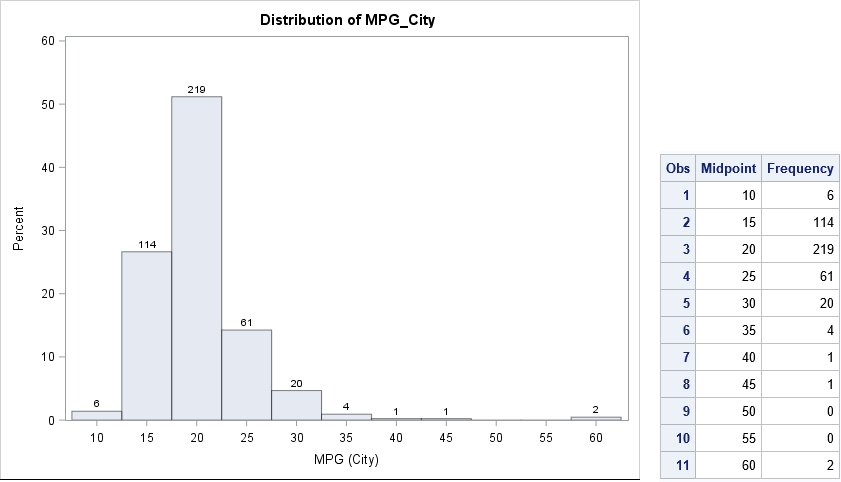### Endpoints versus midpoints

As I've previously discussed, PROC UNIVARIATE supports two options for specifying the locations of bins. The MIDPOINTS option specifies that "nice" numbers (for example, multiples of 2, 5, or 10) are used for the midpoints of the bins; the ENDPOINTS option specifies that nice numbers are used for the endpoints of the bins; By default, midpoints are used, as shown in the previous section. The following call to PROC UNIVARIATE uses the ENDPOINTS option and writes the new bin counts to a data set. The histogram is not shown.

```proc univariate data=Sashelp.Cars noprint; var MPG_City; histogram MPG_City / barlabel=count endpoints outhist=EndPtOut; run;   proc print data=EndPtOut; label _MINPT_ = "Left Endpoint" _COUNT_="Frequency"; var _MINPT_ _COUNT_; run;```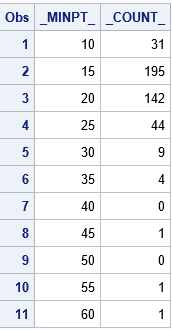### Tabulating counts in the SAS/IML language

If you want to "manually" count the number of observations in each bin, you have a few choices. If you already know the bin width and anchor position for the bins, then you can use a DATA step array to accumulate the counts. You can also use PROC FORMAT to define a format to bin the observations and use PROC FREQ to tabulate the counts.

The harder problem is when you do not have a prior set of "nice" values to use as the endpoints of bins. It is usually not satisfactory to use the minimum and maximum data values as endpoints of the binning intervals because that might result in intervals whose endpoints are long decimal values such as [3.4546667 4.0108333].

Fortunately, the SAS/IML language provides the GSCALE subroutine, which computes "nice" values from a vector of data and the number of bins. The GSCALE routine returns a three-element vector. The first element is the minimum value of the leftmost interval, the second element is the maximum value of the rightmost interval, and the third element is the bin width. For example, the following SAS/IML statements compute nice intervals for the data in the MPG_City variable:

```proc iml; use Sashelp.Cars; read all var "MPG_City" into X; close;   /* GSCALE subroutine computes "nice" tick values: s<=min(x); s>=max(x) */ call gscale(s, x, 10); /* ask for about 10 intervals */ print s[rowname={"Start" "Stop" "Increment"}];```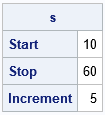The output from the GSCALE subroutine suggests that a good set of intervals to use for binning the data are [10, 15), [15, 20), ..., [55, 60]. These are the same endpoints that are generated by using the ENDPOINTS option in PROC UNIVARIATE. (Actually, the procedure uses half-open intervals for all bins, so it adds the extra interval [60, 65) to the histogram.)

I've previously shown how to use the BIN and TABULATE functions in SAS/IML to count the observations in a set of bins. The following statements use the values from the GSCALE routine to form evenly spaced cutpoints for the binning:

```cutPoints = do(s, s, s); /* use "nice" cutpoints from GSCALE */ *cutPoints = do(s, s+s, s); /* ALTERNATIVE: add additional cutpoint to match UNIVARIATE */ b = bin(x, cutPoints); /* find bin for each obs */ call tabulate(bins, freq, b); /* count how many obs in each bin */ binLabels = char(cutPoints[bins]); /* use left endpoint as labels for bins */ print freq[colname = binLabels label="Count"];```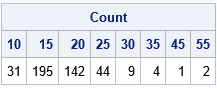Except for the last interval, the counts are the same as for the ENDPOINTS option in PROC UNIVARIATE. It is a matter of personal preference whether you want to treat the last interval as a closed interval or whether you want all intervals to be half open. If you want to exactly match PROC UNIVARIATE, you can modify the definition of the cutPoints variable, as indicated in the program comments.

Notice that the TABULATE routine only reports the bins that have nonzero counts. If you prefer to obtain counts for ALL bins—even bins with zero counts—you can use the TabulateLevels module, which I described in a previous blog post.

In summary, you can use PROC UNIVARIATE or SAS/IML to create a tabular representation of a histogram. Both procedures provide a way to obtain "nice" values for the bin endpoints. If you already know the endpoints for the bins, you can use other techniques in SAS to produce the table.

The post When is a histogram not a histogram? When it's a table! appeared first on The DO Loop.I remember the first time I used PROC GLM in SAS to include a classification effect in a regression model. I thought I had done something wrong because the parameter estimates table was followed by a scary-looking note:

```Note: The X'X matrix has been found to be singular, and a generalized inverse
was used to solve the normal equations. Terms whose estimates are
followed by the letter 'B' are not uniquely estimable.
```

Singular matrix? Generalized inverse? Estimates not unique? "What's going on?" I thought.

In spite of the ominous note, nothing is wrong. The note merely tells you that the GLM procedure has computed one particular estimate; other valid estimates also exist. This article explains what the note means in terms of the matrix computations that are used to estimate the parameters in a linear regression model.

### The GLM parameterization is a singular parameterization

The note is caused by the fact that the GLM model includes a classification variable. Recall that a classification variable in a regression model is a categorical variable whose levels are used as explanatory variables. Examples of classification variables (called CLASS variables in SAS) are gender, race, and treatment. The levels of the categorical variable are encoded in the columns of a design matrix. The columns are often called dummy variables. The design matrix is used to form the "normal equations" for least squares regression. In terms of matrices, the normal equations are written as (X`*X)*b = X`*Y, where X is a design matrix, Y is the vector of observed responses, and b is the vector of parameter estimates, which must be computed.

There are many ways to construct a design matrix from classification variables. If X is a design matrix that has linearly dependent columns, the crossproduct matrix X`X is singular. Some ways of creating the design matrix always result in linearly dependent columns; these constructions are said to use a singular parameterization.

The simplest and most common parameterization encodes each level of a categorical variable by using a binary indicator column. This is known as the GLM parameterization. It is a singular parameterization because if X1, X2, ..., Xk are the binary columns that indicate the k levels, then Σ Xi = 1 for each observation.

Not surprisingly, the GLM procedure in SAS uses the GLM parameterization. Here is an example that generates the "scary" note. The data are a subset of the Sashelp.Heart data set. The levels of the BP_Status variable are "Optimal", "Normal", and "High":

```data Patients; set Sashelp.Heart; keep BP_Status Cholesterol; if ^cmiss(BP_Status, Cholesterol); /* discard any missing values */ run;   proc glm data=Patients plots=none; class BP_Status; model Cholesterol = BP_Status / solution; quit;```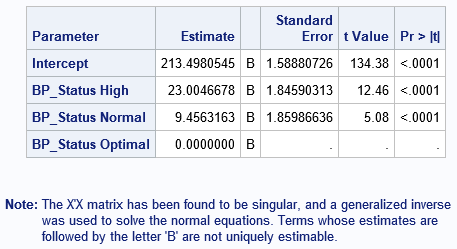### If you change the reference levels, you get a different estimate

If you have linearly dependent columns among the explanatory variables, the parameter estimates are not unique. The easiest way to see this is to change the reference level for a classification variable. In PROC GLM, you can use the REF=FIRST or REF=LAST option on the CLASS statement to change the reference level. However, the following example uses PROC GLMSELECT (without variable selection) because you can simultaneously use the OUTDESIGN= option to write the design matrix to a SAS data set. The first call writes the design matrix that PROC GLM uses (internally) for the default reference levels. The second call writes the design matrix for an alternate reference level:

```/* GLMSELECT can fit the data and output a design matrix in one step */ title "Estimates for GLM Paremeterization"; title2 "Default (Last) Reference Levels"; ods select ParameterEstimates(persist); proc glmselect data=Patients outdesign(fullmodel)=GLMDesign1; class BP_Status; model Cholesterol = BP_Status / selection=none; quit;   /* Change reference levels. Different reference levels result in different estimates. */ title2 "Custom Reference Levels"; proc glmselect data=Patients outdesign(fullmodel)=GLMDesign2; class BP_Status(ref='Normal'); model Cholesterol = BP_Status / selection=none; quit; ods select all;   /* compare a few rows of the design matrices */ proc print data=GLMDesign1(obs=10 drop=Cholesterol); run; proc print data=GLMDesign2(obs=10 drop=Cholesterol); run;```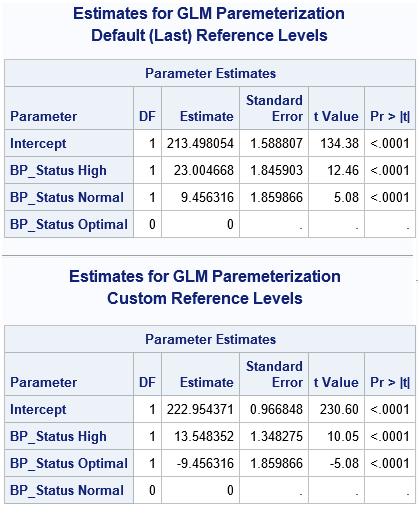The output shows that changing the reference level results in different parameter estimates. (However, the predicted values are identical for the two estimates.) If you use PROC PRINT to display the first few observations in each design matrix, you can see that the matrices are the same except for the order of two columns. Thus, if you have linearly dependent columns, the GLM estimates might depend on the order of the columns.

### The SWEEP operator produces a generalized inverse that is not unique

You might wonder why the parameter estimates change when you change reference levels (or, equivalently, change the order of the columns in the design matrix). The mathematical answer is that there is a whole family of solutions that satisfy the (singular) regression equations, and from among the infinitely many solutions, the GLM procedure chooses the solution for which the estimate of the reference level is exactly zero.

Last week I discussed generalized inverses, including the SWEEP operator and the Moore-Penrose inverse. The SWEEP operator is used by PROC GLM to obtain the parameter estimates. The SWEEP operator produces a generalized inverse that is not unique. In particular, the SWEEP operator computes a generalized inverse that depends on the order of the columns in the design matrix.

The following SAS/IML statements read in the design matrices for each GLM parameterization and use the SWEEP function to reproduce the parameter estimates that are computed by the GLM procedure. For each design matrix, the program computes solutions to the normal equations (X`*X)*b = (X`*Y). The program also computes the Moore-Penrose solution for each design matrix.

```proc iml; /* read design matrix and form X`X and X`*Y */ use GLMDesign1; read all var _NUM_ into Z[c=varNames]; close; p = ncol(Z); X = Z[, 1:(p-1)]; Y = Z[, p]; vNames = varNames[,1:(p-1)]; A = X`*X; c = X`*Y;   /* obtain G2 and Moore-Penrose solution for this design matrix */ Sweep1 = sweep(A)*c; GInv1 = ginv(A)*c; print Sweep1[r=vNames], GInv1;   /* read other design matrix and form X`X and X`*Y */ use GLMDesign2; read all var _NUM_ into Z[c=varNames]; close; p = ncol(Z); X = Z[, 1:(p-1)]; Y = Z[, p]; vNames = varNames[,1:(p-1)]; A = X`*X; c = X`*Y;   /* obtain G2 and Moore-Penrose solution for this design matrix */ Sweep2 = sweep(A)*c; GInv2 = ginv(A)*c; print Sweep2[r=vNames], GInv2;```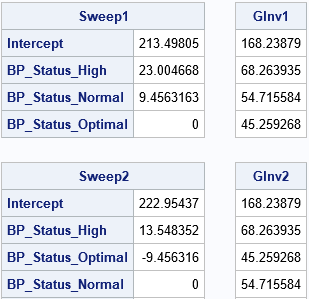The results demonstrate that the SWEEP solution depends on the order of columns in a linearly dependent design matrix. However, the Moore-Penrose solution does not depend on the order. The Moore-Penrose solution is the same no matter which reference levels you choose for the GLM parameterization of classification effects.

In summary, the scary note that PROC GLM produces reminds you of the following mathematical facts:

• When you include classification effects in a linear regression model and use the GLM parameterization to construct the design matrix, the design matrix has linearly dependent columns.
• The X`X matrix is singular when X has linearly dependent columns. Consequently, the parameter estimates for least squares regression are not unique.
• From among the infinitely many solutions to the normal equations, the solution that PROC GLM (and other SAS procedures) computes is based on a generalized inverse that is computed by using the SWEEP operator.
• The solution obtained by the SWEEP operator depends on the reference levels for the CLASS variables.

If the last fact bothers you (it shouldn't), an alternative estimate is available by using the GINV function to compute the Moore-Penrose inverse. The corresponding estimate is unique and does not depend on the reference level.

The post Singular parameterizations, generalized inverses, and regression estimates appeared first on The DO Loop.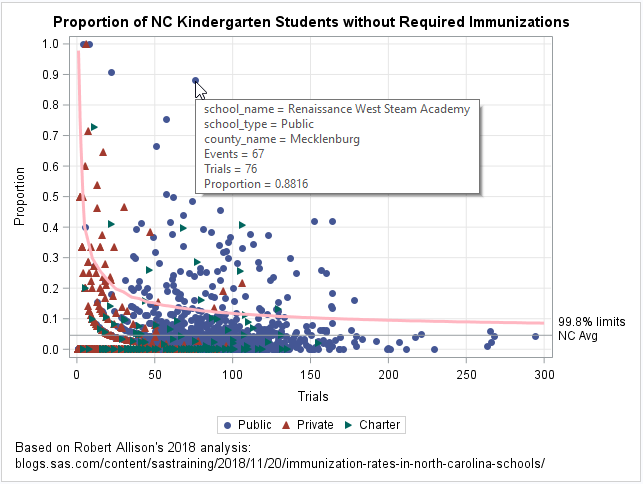Last week my colleague, Robert Allison, visualized data regarding immunization rates for kindergarten classes in North Carolina. One of his graphs was a scatter plot that displayed the proportion of unimmunized students versus the size of the class for 1,885 kindergarten classes in NC. This scatter plot is the basis for a statistical plot that is known as a funnel plot for proportions. The plot to the right shows a funnel plot that I created based on Robert's analysis.

The basic idea of a funnel plot is that small random samples have more variation than large random samples from the same population. If students are randomly chosen from a population in which some small proportion of children have an attribute, it might not be unusual if 40% of the students in a five-student class have the attribute (that's 2 out of 5) whereas it might be highly unusual to see such a high proportion in a 100-student class. The funnel plot enhances a scatter plot by adding curves that indicate a reasonable range of values for the proportion, given the size of a random sample.

For a discussion of funnel plots and how to create a funnel plot in SAS, see the article "Funnel plots for proportions." You can download the immunization data and the SAS program that I used to create the funnel plot for proportions.

### A funnel plot for proportions

The preceding funnel plot contains the following statistical features:
• A scatter plot of the data. Each marker represents a school.
• A horizontal reference line. The line indicates the average proportion for the data. For these data, the statewide average proportion of unimmunized kindergarteners is 4.58%.
• A curve that indicates an upper confidence limit for the proportion of unimmunized students, assuming that the classes are a random sample from a population in which 4.58% of the students are unimmunized. When a marker (school) appears above the curve, the proportion of unimmunized students in that school is significantly higher than the statewide proportion.

This funnel plot uses the shape (and color) of a marker to indicate whether the school is public (circle), private (upward-pointing triangle), or a charter school (right-pointing triangle). The plot includes tool tips so that you can hover the mouse over a marker and see the name and county of the school.

The graph indicates that there are dozens of schools for which the proportion of unimmunized students far exceeds the state average. A graph like this enables school administrators and public health officials to identify schools that have a larger-than-expected proportion of unimmunized students. Identifying schools is the first step to developing initiatives that can improve the immunization rate in school-age children.

### Funnel plots for each school district

You can use a WHERE statement or BY-group processing in PROC SGPLOT to create a funnel plot for each county. A graph that shows only the schools in a particular district is more relevant for local school boards and administrators. The following graphs show the proportion of unimmunized students in Mecklenburg County (near Charlotte) and Wake County (near Raleigh), which are the two largest school districts in NC.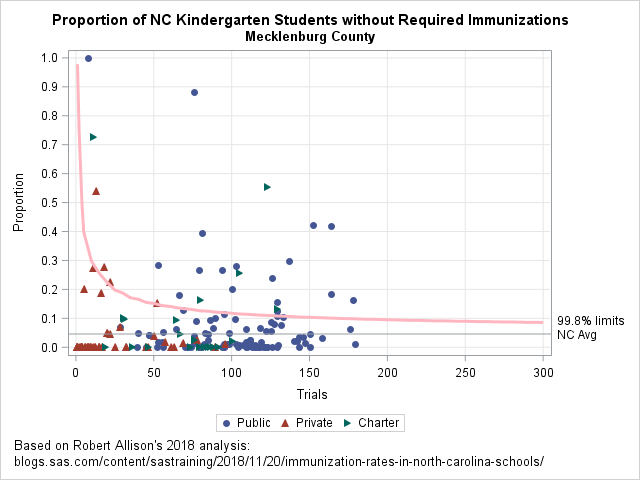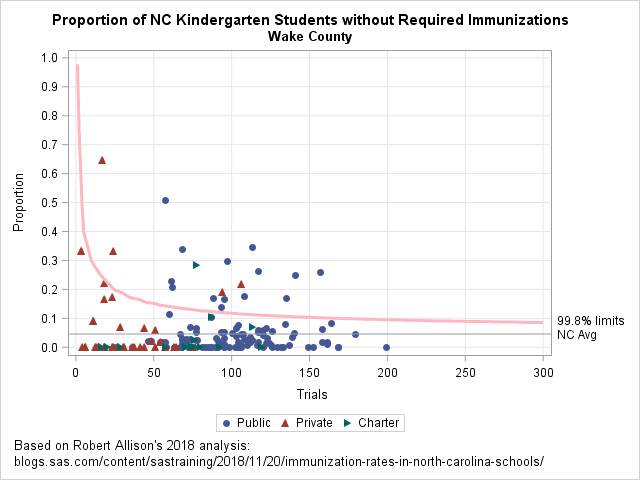The first graph shows that Mecklenburg County has several schools that contain more than 60 kindergarten students and for which 25% or more of the students are unimmunized. In fact, some large schools have more than 40% unimmunized! In Wake County, fewer schools have extreme proportions, but there are still many schools for which the proportion of unimmunized students is quite large relative to the statewide average.

As Robert pointed out in his blog post, these are not official figures, so it is possible that some of the extreme proportions are caused by data entry errors rather than by hordes of unimmunized students.

### Funnel plots for public, private, and charter schools

The following graph shows a panel of plots for public, private, and charter schools. There are many public schools whose proportion of unimmunized students is well above the statewide average. For the private and charter schools, about 10 schools stand out in each group.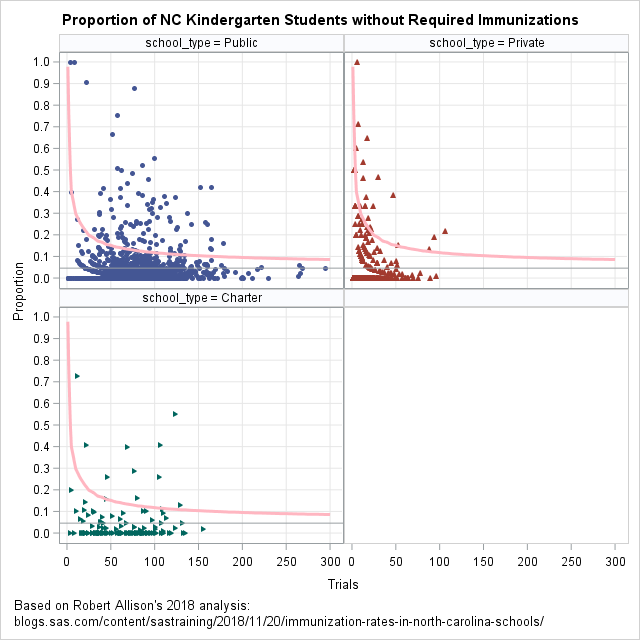I think the plot of private schools is particularly interesting. When the media reports on outbreaks of childhood diseases in schools, there is often a mention of a "religious exemption," which is when a parent or guardian states that a child will not be immunized because of their religious beliefs. The report often mentions that private schools are often affiliated with a particular religion or church. I've therefore assumed that private schools have a larger proportion of unimmunized students because of the religious exemption. These data do not indicate which students are unimmunized because of a religious exemption, but the panel of funnel plots indicates that, overall, not many private schools have an abnormally large proportion of unimmunized students. In fact, the private schools show smaller deviations from the expected value than the public and charter schools.

### Summary

In summary, I started with one of Robert Allison's graphs and augmented it to create a funnel plot for proportions. A funnel plot shows the average proportion and confidence limits for proportions (given the sample size). If the students in the schools were randomly sampled from a population where 4.58% of students are unimmunized, then few schools would be outside of the confidence curve. Of course, in reality, schools are not random samples. Many features of school performance—including unimmunized students—depend on local socioeconomic conditions. By taking into account the size of the classes, the funnel plot identifies schools that have an exceptionally large proportion of unimmunized students. A funnel plot can help school administrators and public health officials identify schools that might benefit from intervention programs and additional resources, or for which the data were incorrectly entered.

The post A funnel plot for immunization rates appeared first on The DO Loop.